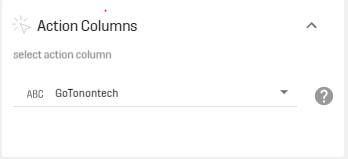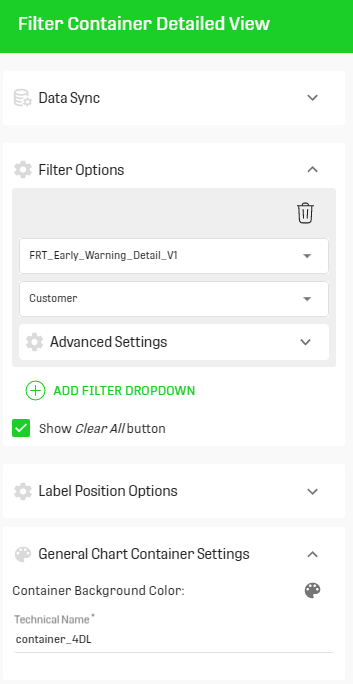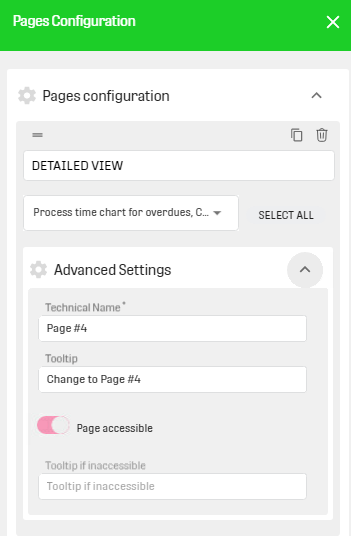## SQL statement for classification evaluation

`SELECT *, case when ISERROR =1 and ISERROR_FORECAST = 1 then '1' when ISERROR = 0 and ISERROR_FORECAST = 1 then 'fp' when ISERROR = 0 and ISERROR_FORECAST = 0 then '0' else 'fn' end as result FROM inputTable`

## Calculate the duration in month between two timestamp columns

```Double Query:
SELECT cast(minNlTs as int) minNltlong, cast(minOrderTs as int) minOrderTslong FROM inputTable
Query:
SELECT (minOrderTslong-minNltlong)/(86400*(365/12)) duration_month FROM inputTable```

## Building hierarchical data

`SELECT f.*, COALESCE(t.SHEET_GUID, s.SHEET_GUID, f.SHEET_GUID) SHEET_GUID  FROM inputTable f left join inputTable s on f.PARENT_GUID = s.GUID left join inputTable t on s.PARENT_GUID = t.GUID `

## Compute a weighted average in SQL

```SELECT index, abs(1/(MaxIndex - index)) AS weights FROM inputTable WHERE index <> MaxIndex
SELECT f.*, s.weights FROM firstInputTable f LEFT JOIN secondInputTable s ON f.index = s.index +1 ORDER BY shipToParty, deliveryDate```

## Cast a string column to a timestamp and then to an integer value

`SELECT cast((unix_timestamp(column)) as int) AS column_ts FROM inputTable`

## Workaround for Date Time Picker and Timestamp use in Reports

`SELECT * FROM inputTable i WHERE  cast(i.due  as int) <= (@due_to@ / 1000)`

## Create a simple lag (Lag t-1) for non equidistant time series in a panel data context

`SELECT f.*, COALESCE(s.deliveryDate, '1900-01-01 00:00:00') AS lastDelivery, COALESCE(s.actualQuantityDelivered, -99) AS ConsumeL1, COALESCE(s.sumOrderQuantity, -99) AS OrderL1, COALESCE(s.freeRiders, -99) AS freeRiderL1 from firstInputTable f LEFT JOIN secondInputTable s ON f.index = s.index +1 `

## Create a binary column 0/1 based on the values in another column (here: actualQuantityDelivered)

`SELECT *, CASE WHEN actualQuantityDelivered = 0 THEN 1 else 0 end as Null_Delivery FROM inputTable ORDER BY index`

## Using LPAD() and RPAD() to bring values to the same length (e.g. fill up with zeroes, etc.)

```SELECT *, LPAD(FRSHTT, 6, '0') AS FRSHTT_new from inputTable

SELECT *, RPAD(FRSHTT, 6, '0') AS FRSHTT_new from inputTable```

## Compare to datetimes on base of the month in a Query, without creating new columns and losing the datetime format of the orginal columns because of stringify

`WHERE substring((CAST (DATUM_MONTAGE_BEGINN AS varchar)),1,7) <= substring((CAST (BUCHUNGSDATUM AS varchar)),1,7)`

## Calculate the duration in days between a timestamp column and today

`(cast(Current_Timestamp() as int) - cast (Kalendertag0CALDAY0CALDAY as int))/86400`

## How to add a day to a timestamp

`SELECT cast(cast(Ende as int) +  86400 as Timestamp) From inputTable`
`SELECT date_add(Column start, int days) AS XYZ FROM inputTable i`

## How to convert a timestamp from UTC to CET timezone

`from_utc_timestamp(CurrentTime, 'CET') AS CurrentTime`

## Create a grouped index using SQL (Index for each group ordered by some variable)

`SELECT *, SUM(Flag) OVER (PARTITION BY IP ORDER BY Index) as cumsum from inputTable`

Input:Result: Index per IP which counts up as soon as the flag column has value "1"## Getting only duplicated values (=values which appear more than one in a column)

Example:```SELECT f.SYSID, f.INSTNO, f.MEASURESTATUS, s.dupeCount
FROM inputTable f
INNER JOIN (
SELECT SYSID, INSTNO, COUNT(*) AS dupeCount
FROM inputTable
GROUP BY SYSID, INSTNO
HAVING COUNT(*) > 1
) s on f.SYSID = s.SYSID AND f.INSTNO = s.INSTNO
ORDER BY f.SYSID, f.INSTNO```

## Split up delimited values in one "cell" into several rows

`SELECT  * , explode(split( XML  , 'DUMMY')) AS NEW FROM inputTable`

The 'DUMMY' can stand for any delimiter, e.g. ",", "/", ...

Example:

Input       A - C - 3/4/5

Output:     A - C - 3

A - C - 4

A - C - 5

## Return the size of delimited values in one "cell"

`CAST(size(split( BUSINESS_AREA_NEW, 'DUMMY')  ) AS double)`

The 'DUMMY' can stand for any delimiter, e.g. ",", "/", ...

Example:

Input:        A - C - 3/4/5/6

Output:     A - C - 4

## Reverse transformation of "Explode + Split" using concat_ws, collect_set, and sort_array(to order the rows in column "col")

`SELECT userid, concat_ws(",", sort_array(collect_set(col))) AS combined FROM inputTable GROUP BY userid`

## Calculate variance, standard deviation etc. in SQL

`Select VARIANCE(QUANTITY) VAR, IDENTIFIER from inputTable i GROUP BY IDENTIFIER`

Example:

Input:

userid - col
1 - a
1 - b
2 - c
2 - d
3 - e

Output:

userid - combined
1 - b,a
2 - c,d
3 - e

## Calculate the median in SQL using PERCENTILE('COLUMN' ,0.5)"

```SELECT variant as variantsCombined,
round(MIN (i.totalThrouputTimeSeconds) /86400,1) AS totalThrouputTimeDaysMINvariantsCombined,
round(AVG (i.totalThrouputTimeSeconds) /86400,1) AS totalThrouputTimeDaysAVGvariantsCombined,
round(MAX (i.totalThrouputTimeSeconds) /86400,1) AS totalThrouputTimeDaysMAXvariantsCombined,
round(PERCENTILE (i.totalThrouputTimeSeconds ,0.5) /86400,1) AS totalThrouputTimeDaysMEDvariantsCombined,
count(variant) AS numberOfVariantsCombined
FROM inputTable i
GROUP BY variant```

## Creating a new column with conditional selection (using sub-queries)

```SELECT *,
CASE WHEN COLUMN_A = 'CLASS_A' THEN Target_value
ELSE (SELECT Target_value FROM inputTable WHERE COLUMN_A = 'CLASS_A')
END AS NEW_COL_NAME
FROM inputTable```

(Nowadays: use the toggle in the respective Join processors!)

```"BroadCastNestedLoopJoins":  SELECT /*+ BROADCAST(secondInputTable) */ * FROM firstInputTable CROSS JOIN secondInputTable

"BroadcastJoins": SELECT /*+ BROADCAST(secondInputTable) */ * FROM firstInputTable  JOIN secondInputTable ON  firstInputTable.joinOnMe = secondInputTable.joinOnMe```

Only use Broadcast Joins when you are sure, that the broadcasted table is small!

## Generating random numbers using the SQL function "RAND()"

RAND() creates a random value between 0 and 1. Any number between the brackets will be used as a seed.

ATTENTION: The seed is then also used in subsequent RAND() functions!

## Extract information from a JSON-object (located within a ONE DATA cell)

Example:Use the SQL function  "get_json_object"

`get_json_object(Activities_and_Sports, '\$.name')`

## Add a cumulative line to a bar chart

Input: Table with column category (e.g. number of pips after dice roll) and column frequency (e.g. number of times a number of pips was diced).

```SELECT
i.category ,
i.frequency ,
SUM( i.frequency ) OVER(ORDER BY i.category ROWS BETWEEN UNBOUNDED PRECEDING AND CURRENT ROW) AS cumulative
FROM inputTable i
ORDER BY i.category```

## Get top 3 (largest entries) per group

Input: Table with a grouping_column (group identifier for which the largest 3 values shall be found) and a value_column which contains the values.

```SELECT * FROM (
SELECT ROW_NUMBER() OVER(PARTITION BY grouping_column ORDER BY value_column DESC) AS Rank, * FROM inputTable)
WHERE Rank <=3```

For smallest values: Order type = ASC in the query

## Avoid e^ conversion in ONE DATA for big numbers

Input: Dataset with numeric column with huge numbers that should be converted to string (right picture shows what happens using the stringify processor)`SELECT CAST(CAST( i.IDENTIFIER as bigint) as string) as IDENTIFIER FROM inputTable `

## Create NaN's with SQL statement (maybe to replace NULL with NaN)

`SELECT COALESCE(column_with_NULLs, CAST('NaN' as double)) AS column_with_NaNs FROM inputTable i`

## Take last 4 characters of a string

`SELECT SUBSTRING(Identifier, LENGTH(Identifier)-3,4) as YEAR FROM inputTable i`

## Get top k results for each group

```SELECT *
FROM (SELECT *, ROW_NUMBER() OVER (PARTITION BY *GroupingColumn* ORDER BY *OrderingColumn* ASC) AS grouped_index FROM inputTable i)
WHERE grouped_index <= *k*```

## Get Json format in FRTs to create links within a table

```SELECT *, CONCAT("{\"label\": \"", COLUMNNAME, "\", ", "\"size\": \"xs\", ", "\"fill-color\": ",  "\"#0000ff\", ", "\"background-color\": \"#ffffff\", ", "\"font-color\": \"#000000\", ","\"partial-fill\": \"0\" ",", \"actions\": [{\"type\":\"page\", \"link\":\"TECHNICAL PAGE NAME\"}, ","{\"type\": \"filter\",\"link\":\"TECHNICAL FILTER CONTAINER NAME\", \"data\": {\"COLUMNNAME\": [\"",COLUMNNAME, "\"]}}]}")
as GoToLink FROM inputTable i```

1. Insert Filterable Result Table in Report
2. Go to settings, → Action Columns
3. Choose column that has been created with json format.Technical Name of Filter Container can be found here: Technical Name of Pages can be found here: